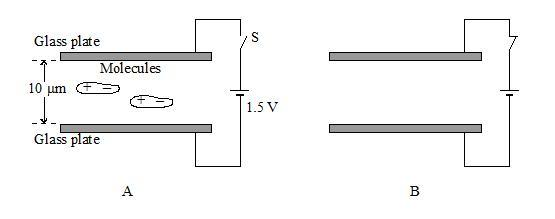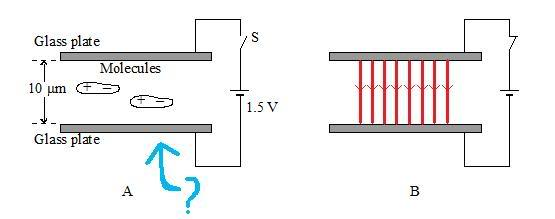# Field, Force, Current

• Air
Once you answer this question, use the same facts to arrive at the answer to the first part.Fleming's LHR is a relation between Current, Force and Magnetic Field. As gendou2 said, how is an Electric Field related to Electric Force [i.e. Coulombic Force]? Once you answer this question, use the same facts to arrive at the answer to the first part.

## Homework Statement

Liquid crystal (LC) displays are found in digital watches and calculators. The display is made from two parallel pieces of glass separated by 1.0 × 10–5m with liquid crystal molecules between them. The glass is coated with conducting material.

The LC molecules have a permanent dipole - that is, they are positive at one end of the molecule and negative at the other. The normal state of these molecules is to be aligned parallel with the glass surfaces as in diagram A. If a voltage of 1.5 V is applied as shown, the molecules align with the electric field.a) On diagram A, show the forces acting on the molecule as the switch S is closed.
b) On diagram B, draw field lines to represent the electric field in the central region of the plates.

2. The attempt at a solutionI drew the field line for part (b), is it correct? Also, I don't know how to draw the force for part (a), how would I show the force? Thanks in advance.

Last edited:
1. Yes, the electric field lines are drawn correctly for the (b) part.

As for the first part, how is Electric Field defined in terms of Electric Force? Once you answer this question, use the same facts to arrive at the answer to the first part.

rohanprabhu said:
As for the first part, how is Electric Field defined in terms of Electric Force? Once you answer this question, use the same facts to arrive at the answer to the first part.

Fleming's LHR? Current, Field and Force perpendicular to each other. I tried this but I couldn't work it out, somehow I got the force to be out of the page.I think the molecules have an electric dipole, not a magnetic dipole.
Fleming's LHR is used for charges moving in a magnetic field.
Fleming's LHR? Current, Field and Force perpendicular to each other. I tried this but I couldn't work it out, somehow I got the force to be out of the page.